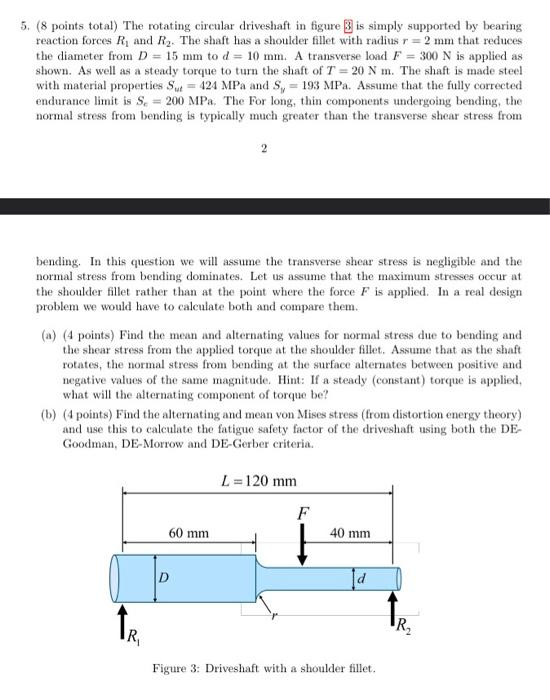# Question 5. (8 points total) The rotating circular driveshaft in figure 3 is simply supported by bearing reaction forces $$R_{1}$$ and $$R_{2}$$. The shaft has a shoulder fillet with radius $$r=2 \mathrm{~mm}$$ that reduces the diameter from $$D=15 \mathrm{~mm}$$ to $$d=10 \mathrm{~mm}$$. A transverse load $$F=300 \mathrm{~N}$$ is applied as shown. As well as a steady torque to turn the shaft of $$T=20 \mathrm{~N}$$. The shaft is made steel with material properties $$S_{u t}=424 \mathrm{MPa}$$ and $$S_{y}=193 \mathrm{MPa}$$. Assume that the fully corrected endurance limit is $$S_{c}=200 \mathrm{MPa}$$. The For long, thin components undergoing bending, the normal stress from bending is typically much greater than the transverse shear stress from 2 bending. In this question we will assume the transverse shear stress is negligible and the normal stress from bending dominates. Let us assume that the maximum stresses occur at the shoulder fillet rather than at the point where the force $$F$$ is applied. In a real design problem we would have to calculate both and compare them. (a) (4 points) Find the mean and alternating values for normal stress due to bending and the shear stress from the applied torque at the shoulder fllet. Assume that as the shaft rotates, the normal stress from bending at the surface alternates between positive and negative values of the same magnitude. Hint: If a steady (constant) torque is applied, what will the alternating component of torque be? (b) (4 points) Find the alternating and mean von Mises stress (from distortion energy theory) and use this to calculate the fatigue safety factor of the driveshaft using both the DEGoodman, DE-Morrow and DE-Gerber criteria. Figure 3: Driveshaft with a shoulder fillet.O8WWPC The Asker · Mechanical EngineeringTranscribed Image Text: 5. (8 points total) The rotating circular driveshaft in figure 3 is simply supported by bearing reaction forces $$R_{1}$$ and $$R_{2}$$. The shaft has a shoulder fillet with radius $$r=2 \mathrm{~mm}$$ that reduces the diameter from $$D=15 \mathrm{~mm}$$ to $$d=10 \mathrm{~mm}$$. A transverse load $$F=300 \mathrm{~N}$$ is applied as shown. As well as a steady torque to turn the shaft of $$T=20 \mathrm{~N}$$. The shaft is made steel with material properties $$S_{u t}=424 \mathrm{MPa}$$ and $$S_{y}=193 \mathrm{MPa}$$. Assume that the fully corrected endurance limit is $$S_{c}=200 \mathrm{MPa}$$. The For long, thin components undergoing bending, the normal stress from bending is typically much greater than the transverse shear stress from 2 bending. In this question we will assume the transverse shear stress is negligible and the normal stress from bending dominates. Let us assume that the maximum stresses occur at the shoulder fillet rather than at the point where the force $$F$$ is applied. In a real design problem we would have to calculate both and compare them. (a) (4 points) Find the mean and alternating values for normal stress due to bending and the shear stress from the applied torque at the shoulder fllet. Assume that as the shaft rotates, the normal stress from bending at the surface alternates between positive and negative values of the same magnitude. Hint: If a steady (constant) torque is applied, what will the alternating component of torque be? (b) (4 points) Find the alternating and mean von Mises stress (from distortion energy theory) and use this to calculate the fatigue safety factor of the driveshaft using both the DEGoodman, DE-Morrow and DE-Gerber criteria. Figure 3: Driveshaft with a shoulder fillet.
More
Transcribed Image Text: 5. (8 points total) The rotating circular driveshaft in figure 3 is simply supported by bearing reaction forces $$R_{1}$$ and $$R_{2}$$. The shaft has a shoulder fillet with radius $$r=2 \mathrm{~mm}$$ that reduces the diameter from $$D=15 \mathrm{~mm}$$ to $$d=10 \mathrm{~mm}$$. A transverse load $$F=300 \mathrm{~N}$$ is applied as shown. As well as a steady torque to turn the shaft of $$T=20 \mathrm{~N}$$. The shaft is made steel with material properties $$S_{u t}=424 \mathrm{MPa}$$ and $$S_{y}=193 \mathrm{MPa}$$. Assume that the fully corrected endurance limit is $$S_{c}=200 \mathrm{MPa}$$. The For long, thin components undergoing bending, the normal stress from bending is typically much greater than the transverse shear stress from 2 bending. In this question we will assume the transverse shear stress is negligible and the normal stress from bending dominates. Let us assume that the maximum stresses occur at the shoulder fillet rather than at the point where the force $$F$$ is applied. In a real design problem we would have to calculate both and compare them. (a) (4 points) Find the mean and alternating values for normal stress due to bending and the shear stress from the applied torque at the shoulder fllet. Assume that as the shaft rotates, the normal stress from bending at the surface alternates between positive and negative values of the same magnitude. Hint: If a steady (constant) torque is applied, what will the alternating component of torque be? (b) (4 points) Find the alternating and mean von Mises stress (from distortion energy theory) and use this to calculate the fatigue safety factor of the driveshaft using both the DEGoodman, DE-Morrow and DE-Gerber criteria. Figure 3: Driveshaft with a shoulder fillet.